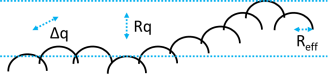## Contacts

### Quick Start

The harder you squeeze two surfaces in contact, the bigger the real contact area (the apparent contact area is irrelevant!) and the higher the electrical and thermal conductivities across the boundary. The effects are more subtle than we might think, depending on the modulus (of course) but also on some very specific roughness measures.

### Credits

The app is inspired by Ch 7 of the excellent book Contact Mechanics and Friction: Physical Principles and Applications by Prof Valentin L Popov, TU Berlin Inst. Mechanik.

### Contacts

Modulus E GPa
Hardness σ0 GPa
F N
A0 cm²
Rq μm
Δq
Reff μm
A μm²
Arel
σrel
Conductivityrel
//One universal basic required here to get things going once loaded
Main();
};
//Any global variables go here

//Main is hard wired as THE place to start calculating when input changes
//It does no calculations itself, it merely sets them up, sends off variables, gets results and, if necessary, plots them.
function Main() {
saveSettings();

//Send all the inputs as a structured object
//If you need to convert to, say, SI units, do it here!
const inputs = {
E: sliders.SlideE.value * 1e9, //From GPa
sigma0: sliders.Slidesigma0.value * 1e9,
F: sliders.SlideF.value,
A0: sliders.SlideA0.value * 1e-4, //cm2 to m2
Rq: sliders.SlideRq.value * 1e-6, //From μm
Dq: sliders.SlideDq.value
}

//Get all the resonses as a structure
const result = CalcIt(inputs)

//Set all the text box outputs
document.getElementById('Reff').value = result.Reff
document.getElementById('A').value = result.A
document.getElementById('Arel').value = result.Arel
document.getElementById('sigmaRel').value = result.sigmaRel
if (result.sigmaRel > 1) {
document.getElementById('sigmaRel').style.backgroundColor = "pink"
} else {
document.getElementById('sigmaRel').style.backgroundColor = document.getElementById('Arel').style.backgroundColor
}
document.getElementById('condRel').value = result.condRel
}

//Here's the real app calculation
function CalcIt({ E, sigma0, F, A0, Rq, Dq }) {
//The structure automatically has the names provided from input
//By convention the values are provided with the correct units within CalcIt
const Reff = Rq / Math.pow(Dq, 2)
const Poisson = 0.3 //The exact value doesn't matter much
const Estar = E / (2 * (1 - Math.pow(Poisson, 2)))
const A = 2 * F / (Estar * Dq)
const sigmaRel = (F / A) / sigma0
const condRel = Math.min(1, 3.7 * F / (Estar * Rq))

return {
Reff: (Reff * 1e6).toPrecision(3),
A: (A * 1e12).toPrecision(3) + "μm²",
Arel: (A / A0).toExponential(3),
sigmaRel: sigmaRel.toPrecision(3),
condRel: condRel.toPrecision(3),
}
}We can characterise a rough surface (and basically all surfaces are rough) via a number of measures which you can find in Surface Profile Explorer. For contact mechanics it is convenient to describe the surface as a series of peaks all with the same radius of curvature Reff and with a range of heights governed by a Gaussian distribution, best captured as the rms deviation in the parameter Rq (sometimes called Rrms and in the book called l). Because Reff is only an intellectual construct and can't be measured directly, a measurement readily found (but, alas, seldom used) is Δq, the rms slope (gradient) which is called ∇z in the book. It turns out that formulae sometimes use Rq only, sometimes Δq only and if we want to go back to the original idea of Reff we can find it via

Δq=sqrt("Rq"/R_"eff"

When we apply a load F over an apparent area A0 the load is actually being applied over an area A due to the roughness peaks and troughs. The irrelevance of A0 to all the calculations is one of those non-intuitive and surprising facts of physics, but it also behind the fact that the frictional force (see Friction) is equally independent of A0. The contact area A can be calculated via the effective modulus E"*"=E/(2(1-ν^2)) where ν is Poisson's ratio, assumed here to be 0.3, using:

A=(2F)/(E"*"Δq)

The relative contact area A_"rel"=A/A_0 is usually a small fraction. Not surprisingly, with the main load being applied to such a small real area, the real stress σ=F/A (rather than the pseudo-stress F/A0) can be large. If it is larger than the hardness σ0 then the calculations here are invalid because we start to have plastic deformation. The ratio of σ to σ0 is calculated and turns red as a warning when the ratio is greater than 1.

### Conductivity

Put two surfaces into contact and their electrical or thermal conductivities will depend (surprisingly) not on the area of contact but its "length". So the conductivity, relative to a perfect contact (i.e. its maximum is 1) is given by:

Conductivity_"rel"=(3.7F)/(E"*"Rq)

So conductivity increases with the force applied, which seems intuitive, decreases with larger modulus (also intuitive) but it is not so obvious that it decreases with the rms roughness Rq.